# Qlik Design Blog

All about product and Qlik solutions: scripting, data modeling, visual design, extensions, best practices, etc.Employee

## Recipe for a Pareto Analysis – Revisited

“Which products contribute to the first 80% of our turnover?”

This type of question is common in all types of business intelligence. I say “type of question” since it appears in many different forms: Sometimes it concerns products, but it can just as well concern any dimension, e.g. customer, supplier, sales person, etc. Further, here the question was about turnover, but it can just as well be e.g. number of support cases, or number of defect deliveries, etc.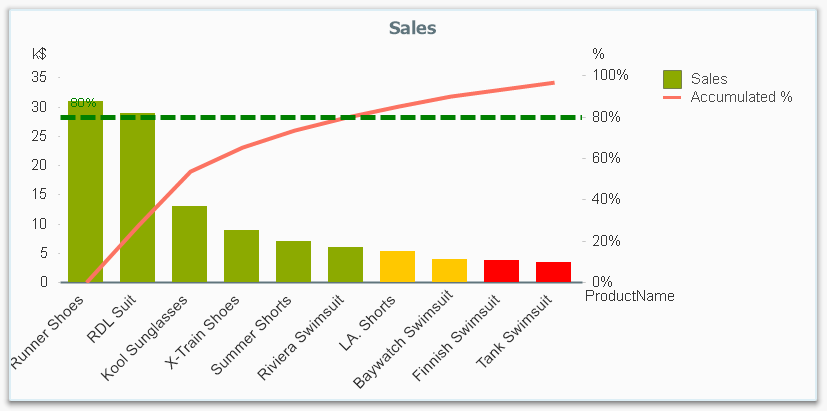It is called Pareto analysis or ABC analysis and I have already written a blog post on this topic. However, in the previous post I only explained how to create a measure which showed the Pareto class. I never showed how to create a dimension based on a Pareto classification – simply because it wasn’t possible.

But now it is.

But first things first. The logic for a Pareto analysis is that you first sort the products according to their sales numbers, then accumulate the numbers, and finally calculate the accumulated measure as a percentage of the total. The products contributing to the first 80% are your best, your “A” products. The next 10% are your “B” products, and the last 10% are your “C” products. In the above graph, these classes are shown as colors on the bars.

The previous post shows how this can be done in a chart measure using the Above() function. However, if you use the same logic, but instead inside a sorted Aggr() function, you can achieve the same thing without relying on the chart sort order. The sorted Aggr() function is a fairly recent innovation, and you can read more about it here.

The sorting is needed to calculate the proper accumulated percentages, which will give you the Pareto classes. So if you want to classify your products, the new expression to use is

=Aggr(
If(Rangesum(Above(Sum({1} Sales)/Sum({1} total Sales),1,RowNo()))<0.8, 'A',
If(Rangesum(Above(Sum({1} Sales)/Sum({1} total Sales),1,RowNo()))<0.9, 'B',
'C')),
(Product,(=Sum({1} Sales),Desc))
)

The first parameter of the Aggr() – the nested If()-functions – is in principle the same as the measure in the previous post. Look there for an explanation.

The second parameter of the Aggr(), the inner dimension, contains the magic of the sorted Aggr():

(Product,(=Sum({1} Sales),Desc))

This structured parameter specifies that the field Product should be used as dimension, and its values should be sorted descending according to Sum({1} Sales). Note the equals sign. This is necessary if you want to sort by expression.

So the Products inside the Aggr() will be sorted descending, and for each Product the accumulated relative sales in percent will be calculated, which in turn is used to determine the Pareto classes.

The set analysis {1} is necessary if you want the classification to be independent of the made selection. Without it, the classification will change every time the selection changes. But perhaps a better alternative is to use {\$<Product=>}. Then a selection in Product (or in the Pareto class itself) will not affect the classification, but all other selections will.

The expression can be used either as dimension in a chart, or in a list box. Below I have used the Pareto class as first dimension in a pivot table.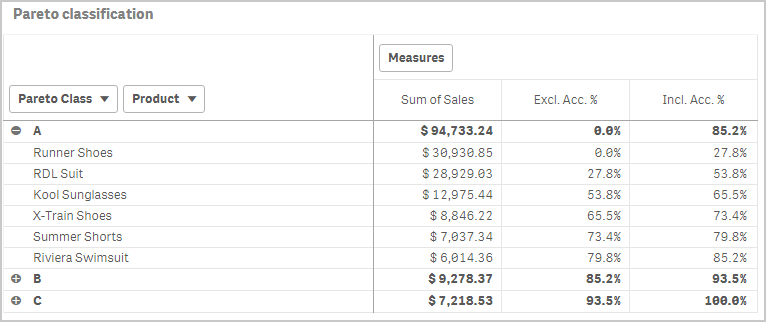If you use this expression in a list box, you can directly select the Pareto class you want to look at.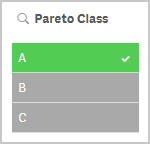The other measures in the pivot table are the exclusive and inclusive accumulated relative sales, respectively. I.e. the lower and upper bounds of the product sales share:

Exclusive accumulated relative sales (lower bound):

=Min(Aggr(
Rangesum(Above(Sum({1} Sales)/Sum({1} total Sales),1,RowNo())),
(Product,(=Sum({1} Sales),Desc))
))

Inclusive accumulated relative sales (upper bound):

=Max(Aggr(
Rangesum(Above(Sum({1} Sales)/Sum({1} total Sales),0,RowNo())),
(Product,(=Sum({1} Sales),Desc))
))

Good luck in creating your Pareto dimension!

HIC

Further reading related to this topic:

The sortable Aggr function is finally here!

Recipe for a Pareto Analysis

Recipe for an ABC Analysis

New Contributor II

Hello Everyone,

As per the discussed logic by hic over the post Recipe for a Pareto Analysis – Revisited‌, want to have a solution over the extended version of the problem which I have mentioned in given below link , So please have a look over it.

Waiting for solution over the given requirement,

Aman

https://community.qlik.com/docs/DOC-19616

914 Views
New Contributor III

Ciao,

i'm encountering an issue with this formula, maybe due to my old 11.2 version?

=Aggr(

If(Rangesum(Above(Sum({1} GIACENZA_ATTUALE*COSTO_MEDIO)/Sum({1} total GIACENZA_ATTUALE*COSTO_MEDIO),1,RowNo()))<0.8, 'A',

If(Rangesum(Above(Sum({1} GIACENZA_ATTUALE*COSTO_MEDIO)/Sum({1} total GIACENZA_ATTUALE*COSTO_MEDIO),1,RowNo()))<0.9, 'B',

'C')),

(COD_ARTICOLO,(=Sum({1} GIACENZA_ATTUALE*COSTO_MEDIO),Desc))

)914 Views
Contributor III

Ciao Fabio,

yes, the problem is QV 11.20, you can't use the same parameter for Aggr function.

QV 11.20

Aggr ([ distinct | nodistinct ] [{set_expression}] expression {, dimension})

QV 12.10

Aggr({SetExpression}[DISTINCT] [NODISTINCT ] expr, StructuredParameter{, StructuredParameter})

Regards

Luca Jonathan Panetta

914 Views
New Contributor III

Ciao,

i have tried this sintax but it wrong.

sorry but i'm not familiar with this type of formulas:

=Aggr(

If(Rangesum(Above(Sum( GIACENZA_ATTUALE*COSTO_MEDIO)/Sum( total

GIACENZA_ATTUALE*COSTO_MEDIO),1,RowNo()))<0.8, 'A',

If(Rangesum(Above(Sum( GIACENZA_ATTUALE*COSTO_MEDIO)/Sum(

total GIACENZA_ATTUALE*COSTO_MEDIO),1,RowNo()))<0.9, 'B',

'C'))

{, COD_ARTICOLO ,(=Sum({1} GIACENZA_ATTUALE*COSTO_MEDIO),Desc)})

Fabio Cuniberto

Da: Luca Jonathan Panetta <qcwebmaster@qlikview.com>

Per: fabio cuniberto <f.cuniberto@arol.it>,

Data: 14/02/2018 09.57

Oggetto: Re: - Recipe for a Pareto Analysis –

Revisited

Recipe for a Pareto Analysis – Revisited

new comment by Luca Jonathan Panetta - View all comments on this blog post

Ciao Fabio,

yes, the problem is QV 11.20, you can't use the same parameter for Aggr

function.

QV 11.20

Aggr ( distinct expression {,

dimension})

QV 12.10

Aggr([DISTINCT] expr, StructuredParameter{,

StructuredParameter})

Regards

Luca Jonathan Panetta

Reply to this email to respond to Luca Jonathan Panetta's comment.

Following Recipe for a Pareto Analysis – Revisited in these streams: Inbox

914 Views
Contributor III

Hi Fabio,

this is yours expression, in bold I mark the possible errors {, ....  }

=Aggr(

If(Rangesum(Above(Sum( GIACENZA_ATTUALE*COSTO_MEDIO)/Sum( total GIACENZA_ATTUALE*COSTO_MEDIO), 1, RowNo() ))<0.8, 'A',

If(Rangesum(Above(Sum( GIACENZA_ATTUALE*COSTO_MEDIO)/Sum(total GIACENZA_ATTUALE*COSTO_MEDIO),1,RowNo()))<0.9, 'B',

'C'))

{, COD_ARTICOLO ,(=Sum({1} GIACENZA_ATTUALE*COSTO_MEDIO),Desc)})

try this

=Aggr(

If(Rangesum(Above(Sum( GIACENZA_ATTUALE*COSTO_MEDIO)/Sum( total GIACENZA_ATTUALE*COSTO_MEDIO), 1, RowNo() ))<0.8, 'A',

If(Rangesum(Above(Sum( GIACENZA_ATTUALE*COSTO_MEDIO)/Sum(total GIACENZA_ATTUALE*COSTO_MEDIO),1,RowNo()))<0.9, 'B',

'C')),

( COD_ARTICOLO, (=Sum({1} GIACENZA_ATTUALE*COSTO_MEDIO), Desc))

)

Regards

Luca Jonathan Panetta

914 Views
New Contributor III

this isn't work in Qlik Sense, would anyone can develop a expression for Qlik sense,

also it would be great if we can add time line for calculation. eg, in a period of sales what's the top80 bottom 20

914 ViewsEmployee

Pareto analysis works fine also in Qlik Sense, as you can see from the screen shots in the blog post. However, if you encounter problems with your expression, I suggest you open a separate threat on the topic.

HIC

914 Views
Contributor

Pareto and Qlik Sense:

Chart Design:

See the comment here by xavier_ch199

Pareto chart in Qlik Sense

To colour code it, using the following expression in the Colour by Expression:

=Aggr(

If(Rangesum(Above(Sum({1} Sales)/Sum({1} total Sales),1,RowNo()))<0.8, 'Green',

If(Rangesum(Above(Sum({1} Sales)/Sum({1} total Sales),1,RowNo()))<0.9, 'Yellow',

'Red')),

(Product,(=Sum({1} Sales),Desc))

)

914 ViewsEmployee

Here is a Waterfall Stacked Barchart with the option of Pareto-like look and feel

http://branch.qlik.com/#!/project/5ab3dc9703e4f8540db95b4d

914 ViewsMVP & Luminary

wow, that's really cool!

914 Views
New Contributor

Hello guys,

I putted as you suggesting but i am having trouble to find the results.

Moreover, how can I turn the pivot table chart into the combined graph as image 1?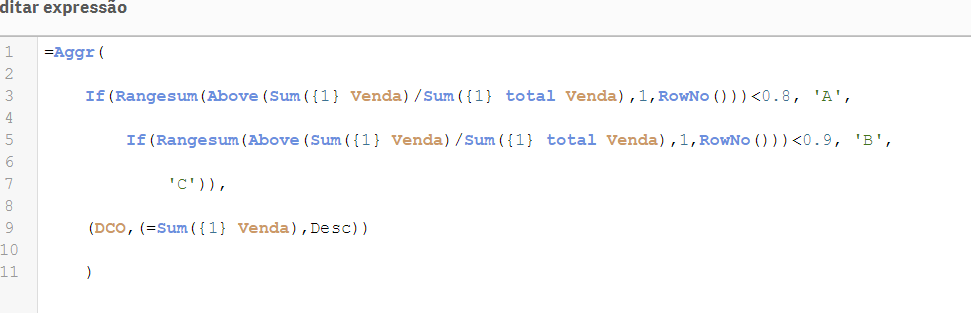914 ViewsEmployee

You have successfully created a Pareto Class as dimension, and it seems to work the way it should. The DCO is not sorted, but that is not really a problem.

However, it sounds as if you want the Pareto Class as a Measure, and then you should not use the Aggr() function. Instead, you should create a measure according to the description on Recipe for a Pareto Analysis. Replace my "Product" field with your "DCO".

Good luck!

HIC

914 Views
New Contributor

Ok! thanks.

Is it possible to put the colours and reference line in the graph?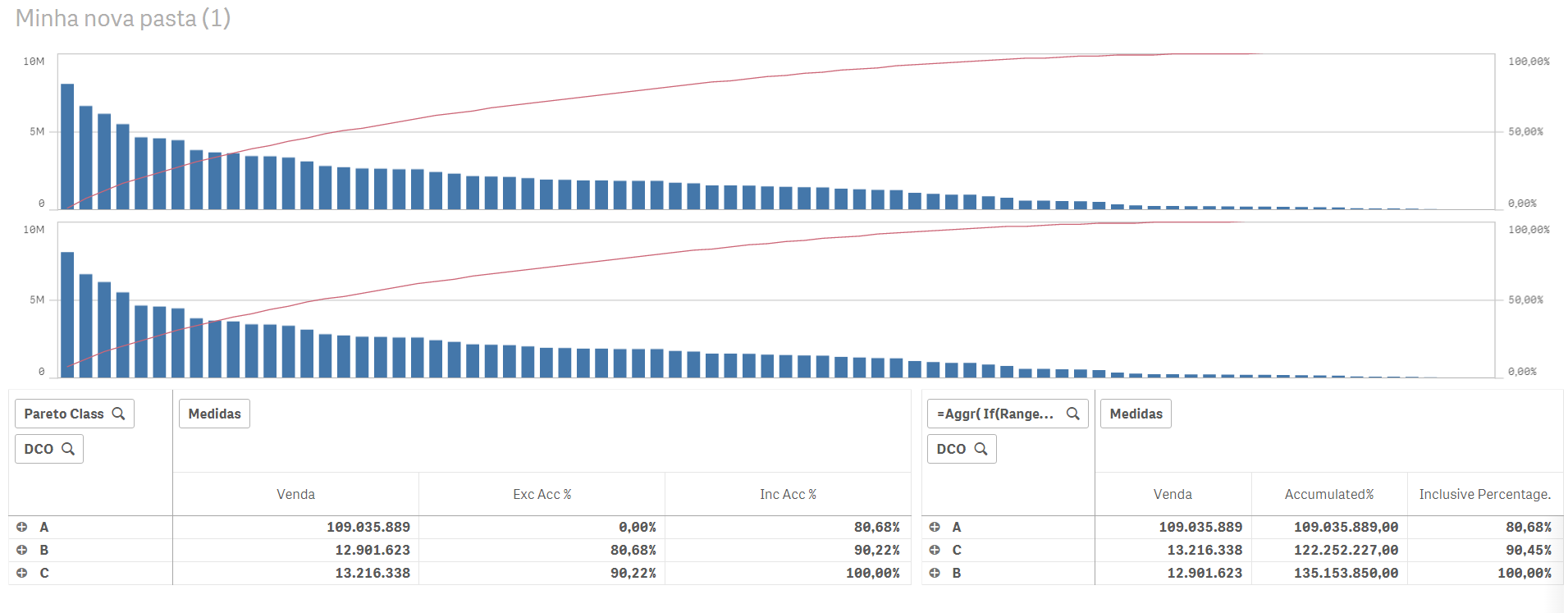914 Views
New Contributor

Sorry forgot to ask again but still learning to use the dashboards:

How can I select only one type of sales: (like in load statement)

sales

from *(QVD)

where

Type = '2018

=RangeSum(Above(Sum(Sales), 0, RowNo())) / Sum(total Sales) //sugested by you

=RangeSum(Above(Sum({\$<Type =  {'2018'} >}  Venda), 0, RowNo())) / Sum(total {\$<Type =  {'2018'} >}  Venda) //trying to convert

same here:

=Aggr(

If(Rangesum(Above(Sum({1} Sales)/Sum({1} total Sales),1,RowNo()))<0.8, 'A',

If(Rangesum(Above(Sum({1} Sales)/Sum({1} total Sales),1,RowNo()))<0.9, 'B',

'C')),

(Product,(=Sum({1} Sales),Desc))

)

914 Views
New Contributor II

Hello,

thank you. It is helpful but how can i choose PARETO CLASS as a parameter?

Thanks.

914 ViewsEmployee

I tried this last week in a POC for a customer using Qlik Sense. Goal was to group customers into A (50% of overall revenue), B (50-95% of overall revenue) and C (the remaining of the overall revenue). Because customer wants to be able to select the "A" or "B" group to further analyze, I have used the formula described in this article to create a calculated dimension. In principle it works well on a relatively small amount of data, however my customer has ~1 million rows in the fact table. With this the calculated dimension is not performant at all. Initial calculation and recalculations take about 90 seconds, which makes it useless for the customer.

Any idea how to make this more performant?

914 ViewsPartner

Hello everyone.

Is it safe to use undocumented feature about sorting by expression in aggr?

777 Views
New Contributor II

Hi,

I want use pareto rule for calculating our top 20 % customers. But when I use formula, it took very long. I have over 200 K customers (rows). Any idea how to solve it?

=Aggr(
If(Rangesum(Above(Sum({1} Sales)/Sum({1} total Sales),1,RowNo()))<0.8, 'A',
If(Rangesum(Above(Sum({1} Sales)/Sum({1} total Sales),1,RowNo()))<0.9, 'B',
'C')),
(customer_id,(=Sum({1} Sales),Desc))
)

733 ViewsPartner

Hello.
You can create a pivot straight table with customer_id as a dimension.
Set sorting by expression Sum({1} Sales) Descending.
Create a measure with label "Cumulative %" and expression
RangeSum(above([Cumulative %]), Sum({1} Sales)/Sum({1} total Sales) ).
Create a measure with expression
If(Rangesum(Above([Cumulative %]))<0.8, 'A',
If(Rangesum(Above([Cumulative %]))<0.9, 'B',
'C')).

I discovered that pivot table has limitations working with interrecord functions, but straight table hasn't.

720 Views
New Contributor II

@abeletsky thanks for suggestion. I did it but something strange happen. It looks like that in the middle of "Cumulative %" column it is restarting RangeSum (see image below). Do you have and idea what could be wrong?

I expect that last row should 100 % in "Cumulative %" column, right?708 ViewsPartner

Hi.

My guess you have not one dimension, so "Cumulative %"  restarts, when above() sees new dimension segment.

Try to add TOTAL in above().

699 ViewsPartner

Hi again.

I think I know why my proposal doesn't work properly.

It is related to "Self-referencing expression".

Quote from Qlik Sense November 2018 help: "Self-referencing expression definitions can only reliably be made in tables with fewer than 100 rows, but this may vary depending on the hardware that the Qlik engine is running on."

https://help.qlik.com/en-US/sense/November2018/Subsystems/Hub/Content/Sense_Hub/ChartFunctions/Inter...

687 Views
New Contributor

ignore

611 Views
New Contributor

Hello,

Only a some questions:

Option #1

1) How can I make the filter of Pareto Class fixed? If I choose A for example it recalculates all over again.

Filter:  =Aggr(
If(Rangesum(Above(Sum({1} Sales)/Sum({1} total Sales),1,RowNo()))<0.5, 'A',
If(Rangesum(Above(Sum({1} Sales)/Sum({1} total Sales),1,RowNo()))<0.8, 'B',
'C')),
([Assortment Group],(=Sum({1} Sales),Desc))
)

Dimension: [Assortment Group]

Measure X: Sum(Sales)

Measure Y (Inclusive Percentage): RangeSum(Above(Sum(Sales), 0, RowNo())) / Sum(total Sales)

2) How can the Y Line does not change the colour?

The Colour Expression that I created was:

If(RangeSum(Above(Sum(Sales),1,RowNo())) / Sum(total Sales) <= 0.5, Green(),
If(RangeSum(Above(Sum(Sales),1,RowNo())) / Sum(total Sales) <= 0.8, Yellow(), ))

Option #2

Filter:  =Aggr(
If(Rangesum(Above(Sum({1} Sales)/Sum({1} total Sales),1,RowNo()))<0.5, 'A',
If(Rangesum(Above(Sum({1} Sales)/Sum({1} total Sales),1,RowNo()))<0.8, 'B',
'C')),
([Assortment Group],(=Sum({1} Sales),Desc))
)

Dimension: [Assortment Group]

Measure X: Sum(Sales)

Measure Y (Inclusive Percentage): RangeSum(Above(Sum(Sales), 0, RowNo())) / Sum(total Sales)

The Colour Expression that I created was:

=Aggr(If(RangeSum(Above(Sum(Sales), 0, RowNo())) / Sum(total Sales))<0.5,Green(),
If(RangeSum(Above(Sum(Sales), 0, RowNo())) / Sum(total Sales)<0.9, Yellow(),Red(),
([Assortment Group],(=Sum({1} Sales),Desc))))

607 Views
New Contributor

Hello all,

i am quite new using Qlik Sense and I have an issue regarding this post Recipe for a Pareto Analysis .

I have done the Pareto analysis creating the master dimension for the ABC classification, but now I would like to create a KPI that indicates, for instance, the number of clients that are 'A', or the sum of sales for the 'A' clients. So I need to use set analysis or a sum (if ...) function but I don't really know whether this is possible or not, and if it is possible I don't know how to do it...

I would really appreciate if someone in the qlik community could help me with this!

Thank you very much in advance

Joan

532 Views
Contributor III

Thanks Henric,

Nice one, for the horizontal axis, is there a way we can show continuous number of suppliers instead, so that the accumulated spend is against of number of suppliers.

268 ViewsPartner

Hello @Henric_Cronström ! Can we use the following syntax in aggr for sorting?  (Product,(=Sum({1} Sales),Desc), (TEXT, ASCENDING))... It looks like it works. Ability to sort by expression is still undocumented. In my case i need to select A, B or C category by pressing a button. So, it should select the same products as it is displayed in a chart. There are some cases when products between A and B categories (or between B and C) have the same values.  In such a cases without using second sorting parameter (TEXT, ASCENDING) aggr function will sort the products by Sales amount and load order... but not by Sales amount and Alphabetical order as it needed in my case...

81 Views